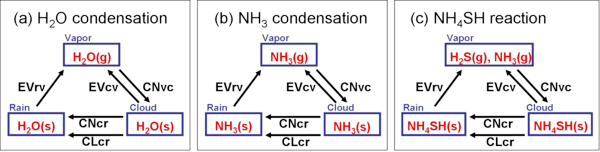### 2.1 Formulation

#### Dynamic framework

The basic equations of the model are based on the quasi-compressible system  and conservation equations of condensible species. For the purpose of facilitating the large horizontal-scale calculations, the model presented here is two-dimensional (2D) in the horizontal and vertical directions only. The details of the dynamic framework are shown in Appendix A.

The numerical code based on this system is, compared to that based on anelastic system employed by Nakajima et al. (2000) , more suitable for large-scale calculation on parallel computers using domain separation, and therefore more suitable for modification from 2D to 3D model, since there is no need to solve elliptic differential equations. The quasi-compressible system is also a useful approximate system which, compared to the full-compressible system, can suppress the increase of computational cost  by substituting the value of sound wave speed to that for the basic reference state. These are the reasons why the quasi-compressible system is often utilized in recent cloud convection models of the Earth's atmosphere.

#### Thermodynamic framework and cloud microphysical model

The model atmosphere consists of H2, He, H2O, NH3, and H2S. The atmosphere is supposed to follow the equation of state of ideal gas. H2 and He are treated as dry (non-condensible) components while H2O, NH3, and H2S are considered as condensible components, and the following condensations and reaction are considered.

• H2O(g) ↔ H2O(s)
• NH3(g) ↔ NH3(s)
• H2S(g) + NH3(g) ↔ NH4SH(s)

The latent and reaction heats are considered in the thermodynamic equation. Each condensed phase species is divided into two categories, ``cloud'' and ``rain''; rain falls down relative to the air, whereas cloud does not. For simplicity, we assume that the condensed phases of cloud and rain consist of pure condensible species; solution is not considered.

The conversion between the categories is implemented by bulk parameterization scheme of Kessler (1969)  used widely in the modeling studies on terrestrial cloud convection. This parameterization scheme evaluates conversion rates between vapor, cloud, and rain by considering the cloud microphysical processes shown in Fig. 2.1. This parameterization is considered to be suited for "warm rain" which does not involve solid phase clouds. It is unclear to what extent the parameterization is approriate to the actual atmosphere of Jupiter, where liquid and solid phase clouds may possibly coexist. In this study, as a starting point, we employ the scheme of Kessler (1969) , selected for its simplicity and wide usage. The details are given in Appendix B.Figure 2.1: Cloud microphysical processes considered in the model. CNvc represents condensation or reaction from vapor to cloud; EVcv, evaporation from cloud to vapor; EVrv, evaporation from rain to vapor; CNcr, autoconversion from cloud to rain; and CLcr, collection from cloud to rain. See Appendix B for details.

#### Turbulent mixing parameterization

The effect of subgrid scale turbulence is implemented using the parameterization scheme of Klemp and Wilhelmson (1978) . The turbulent mixing coefficients are diagnosed from the turbulent kinetic energy that is predicted by a prognostic equation. The details are shown in Appendix C.